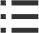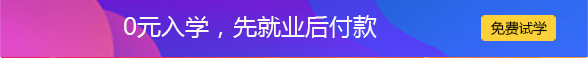2019-09-06 来源：动力节点

Java实现冒泡排序原理

java冒泡排序大概是所有程序员都会用的算法，也是最熟悉的算法之一。

它的思路并不复杂：

设现在要给数组arr[]排序，它有n个元素。

1、如果n=1：显然不用排了。(实际上这个讨论似乎没什么必要)

2、如果n>1：

（1）我们从第一个元素开始，把每两个相邻元素进行比较，如果前面的元素比后面的大，那么在最后的结果里面前者肯定排在后面。所以，我们把这两个元素交换。然后进行下两个相邻的元素的比较。如此直到最后一对元素比较完毕，则第一轮排序完成。可以肯定，最后一个元素一定是数组中最大的(因为每次都把相对大的放到后面了)。

（2）重复上述过程，这次我们无需考虑最后一个，因为它已经排好了。

（3）如此直到只剩一个元素，这个元素一定是最小的，那么我们的排序可以结束了。显然，进行了n-1次排序。

上述过程中，每次(或者叫做“轮”)排序都会有一个数从某个位置慢慢“浮动”到最终的位置(画个示意图，把数组画成竖直的就可以看出来)，就像冒泡一样，所以，它被称为“冒泡排序法”。

代码实现：

public class BubbleSort{

public static void main(String[] args){

int score[] = {67, 69, 75, 87, 89, 90, 99, 100};

for (int i = 0; i < score.length -1; i++){  //最多做n-1趟排序

for(int j = 0 ;j < score.length - i - 1; j++){  //对当前无序区间score[0......length-i-1]进行排序(j的范围很关键，这个范围实在逐步缩小的)

if(score[j] < score[j + 1]){  //把小的值交换到后面

int temp = score[j];

score[j] = score[j + 1];

score[j + 1] = temp;

}

}

System.out.print("第" + (i + 1) + "次排序结果：");

for(int a = 0; a < score.length; a++){

System.out.print(score[a] + " ");

}

System.out.println("");

}

System.out.print("最终排序结果：");

for(int a = 0; a < score.length; a++){

System.out.print(score[a] + " ");

}

}

}

我们忽略掉循环变量自增和初始化的时间。先分析算法的比较次数。容易看出，上面这种未经任何改进的冒泡排序无论输入数据如何都会进行n-1轮排序，而每轮排序需要比较的次数从n-1递减到0。那么，总的比较次数即是 (n-1)+(n-2)+...+2+1 = (n-1)n/2≈(n^2)/2。

再来看下赋值次数。这里的赋值是指其中的交换操作，对于上述代码，1次交换等于三次赋值。由于并非每次都必须交换，因此，赋值操作的次数与输入数据有关。最佳情况(best case)下，即一开始就是有序的情况下，赋值次数为0。 而最坏情况(worst case)下，赋值次数为(n-1)n/2。假设输入数据平均(或者说“完全随机”)分布，那么大约有交换次数为比较次数的一半。由上面的结果，可以得到平均情况(average case)下，赋值次数为 3/2 * (n^2)/2 = 3/4*(n^2).

综上，无论在何种情况下，冒泡排序空间复杂度(额外空间)总是O(1)。

改进

在数据完全有序的时候展现出最优时间复杂度，为O(n)。其他情况下，几乎总是O(n^2)。因此，算法在数据基本有序的情况下，性能最好。

但是，上面的代码怎么可能出现O(n)复杂度呢?实际上，因为上面注重的是基本思路，因此只是最简单情况，要使算法在最佳情况下有O(n)复杂度，需要做一些改进，改进后的代码为：

public static void bubbleSort(int[] arr) {

int temp = 0;

boolean swap;

for (int i = arr.length - 1; i > 0; --i) { // 每次需要排序的长度

swap=false;

for (int j = 0; j < i; ++j) { // 从第一个元素到第i个元素

if (arr[j] > arr[j + 1]) {

temp = arr[j];

arr[j] = arr[j + 1];

arr[j + 1] = temp;

swap=true;

}

}//loop j

if (swap==false){

break;

}

}//loop i

}// method bubbleSort

算法稳定性

容易看出，在相邻元素相等时，我们并不需要交换它们的位置，所以，冒泡排序是稳定排序。

算法适用场景

冒泡排序思路简单，代码也简单，特别适合小数据的排序。但是，由于算法复杂度较高，在数据量大的时候不适合使用。如果一定要在较多数据的时候使用，最好对算法加以改进，例如选择排序法。

java新手入门教程下载之冒泡排序分析：http://www.bjpowernode.com/xiazai/2539.html